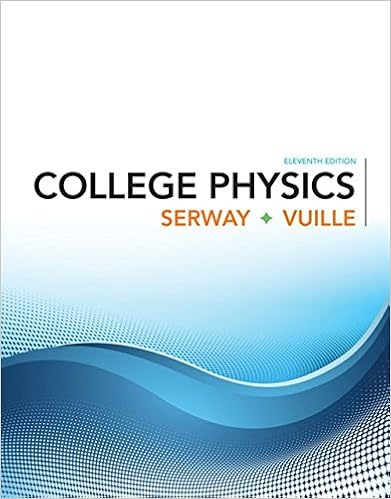# 30 a box is sliding down an incline tilted at an

• Test Prep
• 8
• 100% (10) 10 out of 10 people found this document helpful

This preview shows page 5 - 8 out of 8 pages.

##### We have textbook solutions for you!
The document you are viewing contains questions related to this textbook.The document you are viewing contains questions related to this textbook.
Chapter 3 / Exercise 3.6
College Physics
Serway/VuilleExpert Verified
30) A box is sliding down an incline tilted at an angle 14.0° above horizontal. The box is sliding down the incline at a speed of 1.70 m/s. The coefficient of kinetic friction between the box and the incline is 0.380. How far does the box slide down the incline before coming to rest?
##### We have textbook solutions for you!
The document you are viewing contains questions related to this textbook.The document you are viewing contains questions related to this textbook.
Chapter 3 / Exercise 3.6
College Physics
Serway/VuilleExpert Verified
31) A ball is tied to the end of a cable of negligible mass. The ball is spun in a circle with a radius 2.00 m making 0.700 revolutions per second. What is the centripetal acceleration of the ball? 2
32) A car travels around 200 m radius flat curve at 40 m/s at constant speed. What is the minimum static coefficient of friction which allows it to travel at this speed without sliding?
33) A car drives over a hilltop that has a radius of curvature 120 m at the top of the hill. At what speed would the car be traveling when it tires just barely lose contact with the road when the car is at the top of the hill? A) 45.5 m/s B) 41.8 m/s C) 34.3 m/s D) 22.2 m/s E) 27.6 m/s
34) An airplane is flying with constant speed of 300 m/s along a horizontal circle with a radius of 15,000 m. If the lift force of the air on the wings is perpendicular to the wings, at what angle relative to the horizontal should the wings be banked?
35) A car drives over a hilltop that has a radius of curvature 120 m at the top of the hill. At what speed would the car be traveling when it tires just barely lose contact with the road when the car is at the top of the hill?
ANSWERS 1. B 2. 5.9 × 10 4 3. A 4. E 5. D 6. A 7. B 8. B 9. C 10. B 11. B 12. D 13. C 14. D 15. B 16. E 17. E 18. D 19. B 20. C 21. E 22. A 23. C
•••